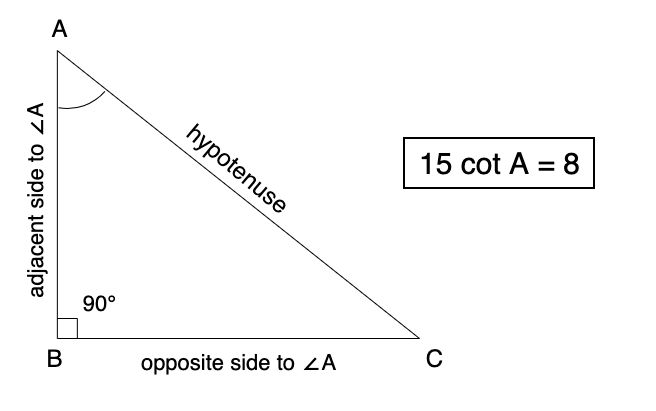# 15 cot A = 8 | Find the value of sin A and sec A | Trigonometry Numerical

Comments

## Question: Given 15 cot A = 8, find sin A and sec A

In problems like these, make sure you know all the formulas mentioned in the notes basics of trigonometry by heart. This will help you gain speed and confidence in solving problems like these.

### Solution:

Consider $\triangle ABC$, where $m \angle B$ is $90 \degree$.fig. 1

\begin{aligned} &15 \sdot \cot \ A = 8 \\ \therefore \ &\cot \ A = {8 \over 15} \quad --- (1)\\ \\ From \ &fig. \ 1, \\ \cot A &= {\text{Adjacent side to } \angle A \over \text{Opposite side to } \angle A} \\ \cot A &= {AB \over BC} \\ \\ Replacing \ &this \ in \ equation \ (1) \\ \therefore \ &{AB \over BC} = {8 \over 15} \quad --- (2) \quad \text{[from figure]} \end{aligned}

From equation (2), we can say that $AB = 8x$ and $BC = 15x$.

Wondering why we have taken "8x" and "15x" here ?

Check this video for understanding it.Applying Pythagoras theorem on $\triangle ABC$ to find the value of $AC$, we get

\begin{aligned} AC^2 &= AB^2 + BC^2 \\ &= (8x)^2 + (15x)^2 \\ &= 64x^2 + 225x^2 \\ \therefore \ AC^2 &= 289x^2 \\ \therefore \ AC^2 &= (17x)^2 \\ \therefore \ AC &= 17x \quad ---(3) \end{aligned}

Now,

\begin{aligned} \sin \ A &= {\text{Opposite side to } \angle A \over \text {Hypotenuse}} \\ \sin \ A &= {BC \over AC} \\ &= {15x \over 17x} \\ &\boxed {\therefore \ \sin \ A = {15 \over 17}} \end{aligned}

\begin{aligned} \sec \ A &= {Hypotenuse \over \text{Adjacent side to } \angle A} \\ &= {AC \over AB} \\ &= {17x \over 8x} \\ &\boxed {\therefore \ \sec \ A = {17 \over 8}} \end{aligned}
Without doing more calculation on paper, can you find the values of tan A, cos A and cosec A? Let us know your answers in comments below and also how you got the values without calculation.

### Comments:

All comments that you add will await moderation. We'll publish all comments that are topic related, and adhere to our Code of Conduct.

Want to tell us something privately? Contact Us